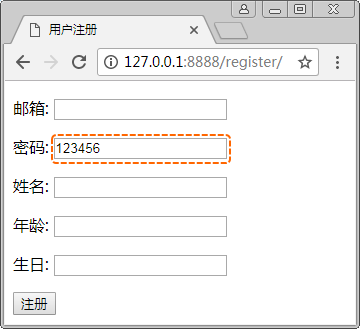﻿ Django2：Web项目开发入门笔记（21） – 魔力Python

# Django2：Web项目开发入门笔记（21）

Django教程 5062浏览

```from django.db import models

class UserModel(models.Model):
email = models.EmailField('邮箱')
name = models.CharField('姓名', max_length=20)
age = models.IntegerField('年龄')
birthday = models.DateField('生日')```

```from django import forms
from FormTest import models  # 导入模型模块

class RegisterForm(forms.ModelForm):  # 继承ModelForm类
class Meta:
model = models.UserModel
fields = '__all__'```

```from django import forms

class RegisterForm(forms.Form):
email = forms.EmailField(label='邮箱')
name = forms.CharField(label='姓名', max_length=20)
age = forms.IntegerField(label='年龄')
birthday = forms.DateField(label='生日')```

Model 字段 Form 字段
AutoField 没有对应的字段
BigAutoField 没有对应的字段
BigIntegerField IntegerField（+-9223372036854775808之间）
BooleanField BooleanField
CharField CharField

max_length=8对应Model字段max_length=8

empty_value=None对应Model字段null=True

DateField DateField
DateTimeField DateTimeField
DecimalField DecimalField
EmailField EmailField
FileField FileField
FilePathField FilePathField
FloatField FloatField
ForeignKey ModelChoiceField
ImageField ImageField
IntegerField IntegerField
ManyToManyField ModelMultipleChoiceField
NullBooleanField NullBooleanField
PositiveIntegerField IntegerField
PositiveSmallIntegerField IntegerField
SlugField SlugField
SmallIntegerField IntegerField
TextField CharField（widget=forms.Textarea）
TimeField TimeField
URLField URLField

```<!DOCTYPE html>
<html lang="zh-cn">
<meta charset="UTF-8">
<title>用户注册</title>
<body>
<form action="/register/" method="post">
{% csrf_token %}
{{ form.as_p }}
<input type="submit" value="注册">
</form>
</body>
</html>```

```from django.urls import path
from FormTest import views as form_views

urlpatterns = [
path('register/', form_views.register),
]```

```from django.shortcuts import render, HttpResponse
from FormTest.forms import RegisterForm

def register(request):
if request.method == 'POST':
form = RegisterForm(request.POST)
if form.is_valid():
try:
form.save()
except:
return HttpResponse('糟糕，注册失败了！！')
return HttpResponse('恭喜您，注册成功！')
form = RegisterForm()
return render(request, 'register.html', {'form': form})``````class RegisterForm(forms.ModelForm):  # 继承ModelForm类
class Meta:
model = models.UserModel
fields = '__all__'
widgets = {
}```

```labels = {
'name': '芳名'
}```

```help_texts = {
'birthday': ('日期格式：2018-2-28'),
}```

```error_messages = {
'name': {
'max_length': ("你那个太长了啦！."),
},
}```

```field_classes = {
'age': forms.CharField
}```

`fields = ['email', 'password', 'age']`

`exclude = ['birthday']`

1、表单字段值修改后，存入数据库。

```from django.shortcuts import render, HttpResponse
from FormTest.forms import RegisterForm
from hashlib import md5

def register(request):
if request.method == 'POST':
form = RegisterForm(request.POST)
if form.is_valid():
email = form.cleaned_data['email']
try:
new_form = form.save(commit=False)
m = md5()
new_form.save()
form.save_m2m()
except:
# raise
return HttpResponse('糟糕，注册失败了！！')
return HttpResponse('恭喜您，注册成功！')
form = RegisterForm()
return render(request, 'register.html', {'form': form})```

2、一些字段无需用户填写，由系统自动给定。

```class RegisterForm(forms.ModelForm):
class Meta:
model = models.UserModel
exclude = ['birthday']```

```...省略部分代码...
from FormTest.models import UserModel

def register(request):
if request.method == 'POST':
user =UserModel(birthday='1990-1-1')
form = RegisterForm(request.POST,instance=user)
if form.is_valid():
...省略部分代码...```

```from django import forms
from FormTest import models
class RegisterForm(forms.ModelForm):
class Meta:
model = models.UserModel

```from django import forms
from FormTest import models

```from django import forms
from FormTest import models

RegisterForm= forms.modelform_factory(models.UserModel,